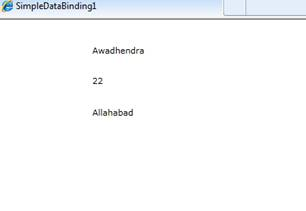In this demonstration we learn concept of simple data binding in Silverlight. As we know data binding means to bind some records with controls. On the basis of controls data binding is of two types, Simple data binding and complex data binding. In simple data binding we use such type of control which can display only one value at a time and while in complex data binding we use such type of control which is able to display more than one value. Best example of simple data binding is TextBlock, Button control etc.

##### Write following XAML code to create an user interface for demonstration
`<Grid x:Name="LayoutRoot" Background="White">        <TextBlock Height="23" HorizontalAlignment="Left" Margin="129,33,0,0" Name="textBlock1" Text="{Binding Name}" VerticalAlignment="Top" Width="157" />        <TextBlock Height="23" HorizontalAlignment="Left" Margin="129,73,0,0" Name="textBlock2" Text="{Binding Age}" VerticalAlignment="Top" Width="157" />        <TextBlock Height="23" HorizontalAlignment="Left" Margin="129,115,0,0" Name="textBlock3" Text="{Binding City}" VerticalAlignment="Top" Width="157" /></Grid>`

Here mention one thing, In Text property of TextBlock control I had written Text=” {Binding Age}” which means we bind value of Name property with TextBlock control. Name property is created in Employee class.

##### Create a class named Employee
`public class Employee{        public string Name { get; set; }        public string Age { get; set; }        public string City { get; set; }}`
##### Write down the following code at the load event of User Control
`private void UserControl_Loaded(object sender,  RoutedEventArgs e){            textBlock1.DataContext = (new Employee() { Name = "Awadhendra"});            textBlock2.DataContext = (new Employee() { Age = "22" });            textBlock3.DataContext = (new Employee() { City = "Allahabad" });}`
##### Save the application and execute it to show the resultModified On Mar-31-2019 04:13:34 PM